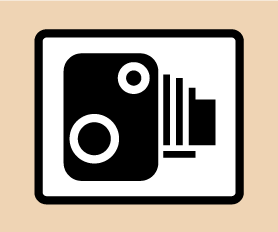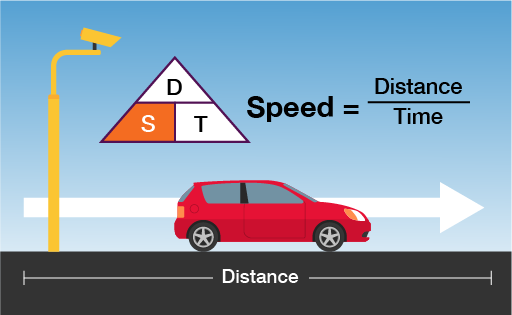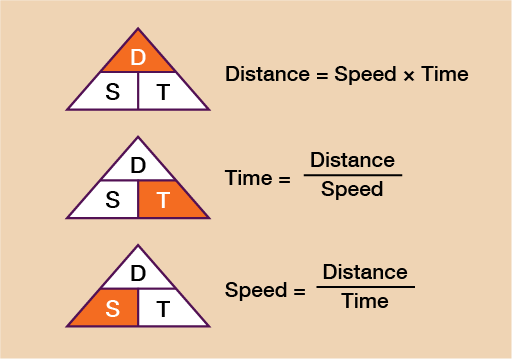Everyday maths 2 (Wales)

Start this free course now. Just create an account and sign in. Enrol and complete the course for a free statement of participation or digital badge if available.

# 3.3 Average speed

The sign below is commonly seen on motorways but it is not the only time when it is useful to know your average speed.Figure 20 A speed camera sign

Being able to calculate and use average speed can help you to work out how long a journey is likely to take. The method for working out average speed involves using a simple formula.Figure 21 A formula for average speed

You can also use this formula to work out the distance travelled when given a time and the average speed, or the time taken for a journey when given the distance and average speed.

The formulas for this are shown in the diagram below. You can see that when given any two of the elements from distance, speed and time, you will be able to work out the third.Figure 22 Distance, speed and time formulas

If you can learn this formula triangle, when you want to use it, you write it down and cover up what you want to work out (the segment in orange). This will tell you what calculation you need to do.

Let’s look at an example of each so that you can familiarise yourself with it.

## Example: Calculating distance

A car has travelled at an average speed of 52 mph over a journey that lasts 2 and a half hours. What is the total distance travelled?

### Method

You can see that to work out the distance you need to do speed × time. In this example then we need to do 52 × 2.5. It is very important to note here that 2 and a half hours must be written as 2.5 (since 0.5 is the decimal equivalent of a half).

You cannot write 2.30 (for 2 hours and 30 mins). If you struggle to work out the decimal part of the number, convert the time into minutes (2 and a half hours = 150 minutes) and then divide by 60 (150 ÷ 60 = 2.5).

52 × 2.5 = 130 miles travelled

## Example: Calculating time

A train will travel a distance of 288 miles at an average speed of 64 mph. How long will it take to complete the journey?

### Method

You can see from the formula that to calculate time you need to do distance ÷ speed so you do:

288 ÷ 64 = 4.5 hours

Again, note that this is not 4 hours 50 minutes but 4 and a half hours.

If you are unsure of how to convert the decimal part of your answer, simply multiply the answer by 60, which will turn it into minutes and you can then convert from there.

In this case, 4.5 × 60 = 270 minutes. We already know from the answer of 4.5 hours that this is 4 whole hours and so many minutes, so we now need to work out how many minutes the .5 represents:

60 × 4 = 240 minutes

270 − 240 = 30 minutes

So 4.5 hours = 270 minutes = 4 hours, 30 minutes

## Example: Calculating speed

A Formula One car covers a distance of 305 km during a race. The time taken to finish the race is 1 hour and 15 minutes. What is the car’s average speed?

### Method

The formula tells you that to calculate speed you must do distance ÷ time. Therefore, you do 305 ÷ 1.25 (since 15 minutes is a quarter of an hour and 0.25 is the decimal equivalent of a quarter):

305 ÷ 1.25 = 244 km/h

In a similar way to example 1, if you are unsure of how to work out the decimal part of the time simply write the time (in this case 1 hour and 15 minutes) in minutes, (1 hour 15 minutes = 75 minutes) and then divide by 60:

75 ÷ 60 = 1.25

Now have a go at the following activity to check that you feel confident with finding speed, distance and time. Please do the calculations first without a calculator. You may then double-check on a calculator if needed.

## Activity 8: Calculating speed, distance and time

1. Filip is driving a bus along a motorway. The speed limit is 70 mph. In 30 minutes, he travels a distance of 36 miles. Does his average speed exceed the speed limit?
2. A plane flies from Frankfurt to Hong Kong. The flight time was 10 hours and 45 minutes. The average speed was 185 km/h. What is the distance flown by the plane?
3. Malio needs to get to a meeting by 11:00 am. The time now is 9:45 am. The distance to the meeting is 50 miles and he will be travelling at an average speed of 37.5 mph. Will he be on time for the meeting?

1. You need to find the speed so you do: distance ÷ time.

The distance is 36 miles. The time is 30 minutes but you need the time in hours:

• 30 minutes ÷ 60 = 0.5 hours

Now you do:

• 36 ÷ 0.5 = 72 mph

Yes, Filip’s average speed did exceed the speed limit.

2. You need to find the distance so you do:
• speed × time
• 10 hours 45 minutes = 10.75 hours

If you are unsure how to express this in hours, convert 10 hours 45 minutes all into minutes:

• 10 × 60 = 600 + 45 = 645 minutes

Then divide by 60:

• 645 ÷ 60 = 10.75 hours

Now to work out the distance do:

• speed × time = 185 × 10.75 = 1988.75 km from Frankfurt to Hong Kong
3. You need to find the time so you do:
• distance ÷ speed
• 50 ÷ 37.5 = 1.33 hours (rounded to two d.p)
• Note: The actual answer is 1.3333333 (the 3 is recurring or never-ending).

To convert this to minutes do:

• 1.33 × 60 = 79.8 minutes
• round 79.8 minutes up to 80 minutes
• 80 minutes = 1 hour and 20 minutes

If the time now is 9:45 am and his meeting is at 11:00 am, then it is only 1 hour, 15 minutes until his meeting, so no, Malio will not make the meeting on time.

Hopefully you will now be feeling more confident with calculations involving speed, distance and time. You will now move on to temperature conversions.

## Summary

In this section you have learned how to:

• use timetables to plan a journey and how to calculate time efficiently
• convert between units of time by using multiplication and division skills
• use the formula for calculating distance, speed and time.#### Find out more

*Eligibility rules apply for financial support.